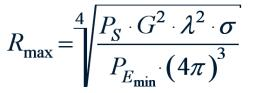# Correctness 3416

Calculate and verify the correctness of the test result:
12 (3c - 5) + 11 = 10 (8c - 7) - 16c

c =  0.75

### Step-by-step explanation:

12(3c - 5) + 11 = 10(8c - 7) - 16c

12·(3·c - 5) + 11 = 10·(8·c - 7) - 16·c

28c = 21

c = 21/28 = 0.75

c = 3/4 = 0.75

Our simple equation calculator calculates it.Did you find an error or inaccuracy? Feel free to write us. Thank you!

Tips for related online calculators
Do you have a linear equation or system of equations and looking for its solution? Or do you have a quadratic equation?